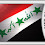## Monday, September 3, 2012

### Problem 802: Triangle, Median, Perpendicular, Angle, Measurement

Geometry Problem
Level: Mathematics Education, High School, Honors Geometry, College.

Click the figure below to see the complete problem 802.1.Prolongar el segmento ED, desde D, 2 unidades hasta el punto que llamamos F, de modo que el segmento EF mide 4 y por tanto el triángulo rectángulo CEF es isósceles, y así el ángulo CFE=45º. Como los triángulos CDF y ADE son congruentes, por ser CD=AD, DF=DE y ángulos opuestos por el vértice en D, se tiene que ángulo AED =àngulo CFD, esto es x=45º.

Migue.

2.tan x
= (AE sin x) / (AE cos x)
= (DC sin CDE) / (DE + DC cos CDE)
= (4) / (2 + 2)
= 1

x = 45 degrees

3.By Pyth. Thm and median, we have AD = DC = sqrt(20) = 2*sqrt(5).
Simply let angle EDC be Y.
Hence, using sine rule,
= 2/(sin(Y-X))
= 2/(sinYcosX - sinXcosY)
Note that sinY = 2/sqrt5 and cosY = 1/sqrt5
=> 2cosX - sinX = sinX
=> cosX = sinX
X = 45.

4.Extend the median to a point P such that AP is perpendicular to EC. Since AD = DC, triangle PAD is congruent to triangle ECD.
tanX = PA/PE = 4/(2+2) = 1, X = 45

1.It should be AP perpendicular to BD, parallel to EC.

2.Yes , thanks Jacob

3.thanks for correction, jacob

5.We draw AP⊥BD
1.ΔAPD= ΔDEC =>ED=DP=2
AP=EC=4
2.Δ APE, AP=4=EP=>angle AED=45º

Another solution
We draw AF⊥CE=>AF‖DF
AF=2ED=4
ΔAEF, angle AEF=45º=>angle AED=45º

ERINA.H NJ

6.So AE is the external angle bisector of angle DEC
and x=1/2.90=45
Peter Tran

7.Draw DF parallel to CE (F on AE)
So FDE = DEC is a right angle
DF = half CE = 2 = DE
cot x = DE/DF = 1
x = 45 deg

8.draw ED and MD=2 then AECM is parall.
AM=ME and M<EMA=90 then x=45

9.Let line perpendicular to AC that passes through A meet BD at P. PA/AD=4/2=PA/(2*sqrt(5)), so PA=4*sqrt(5) while AC=2*(2*sqrt(5))=PA, because sqrt(4^2+2^2)=2*sqrt(5). Then <ACP=45=<AEP.

10.Extend ED to F such that DF=2. Then AECF is a parellogram, EFC is an isoceles right Tr and so x=45

Sumith Peiris
Moratuwa
Sri Lanka

11.Problem 802
Suppose that M is the medium of EC, then EM=2=ED.So <EDM=45.But AD=DC then AE//DM .Therefore x=<AED=<EDM=45.
APOSTOLIS MANOLOUDIS 4 HIGH SHCOOL OF KORYDALLOS PIRAEUS GREECE

12.Problem 802 (sοlution 2)
Let AN//BD ,or AN perpendicular CE (N belongs to the extension of CE).Then NE=NC=4
And AN=2.ED=2.2=4=NE.So <NEA=45=<AED=x.
APOSTOLIS MANOLOUDIS 4 HIGH SHCOOL OF KORYDALLOS PIRAEUS GREECE